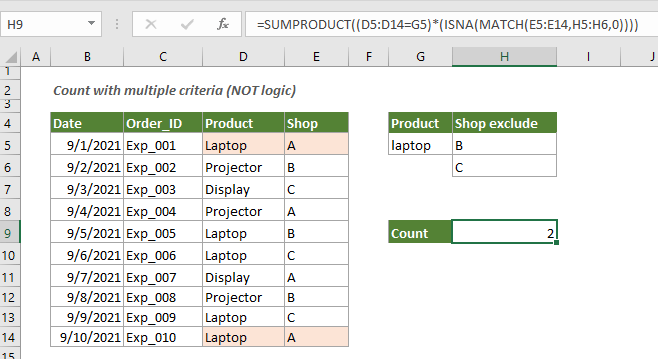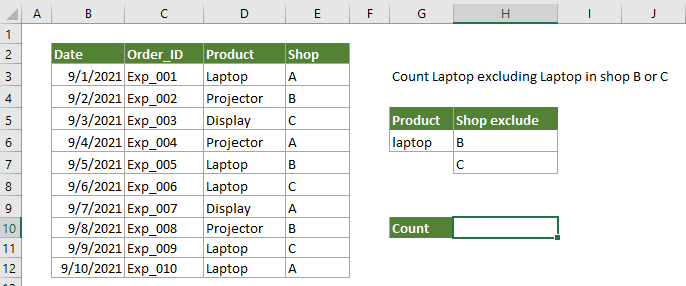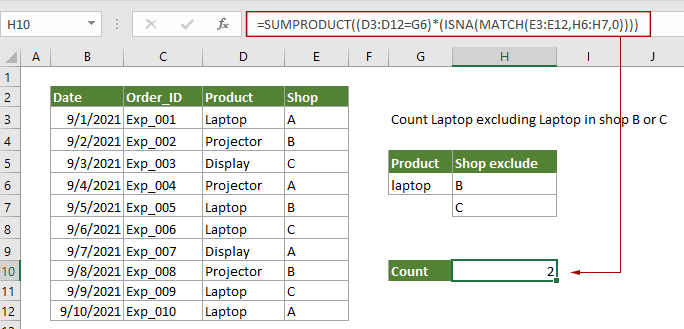## Count multiple criteria with NOT logic in Excel#### How to count multiple criteria with NOT logic in Excel?

As the below product order table shown, to count number of cells that contain Laptop but excluding the laptops in shop B or C, you can apply the below formula to get it done.Generic Formula

=SUMPRODUCT(( range1=criteria1)*(ISNA(MATCH(range2,criteria2,0)))

Arguments

Range1 (required): The range you will count based on the criteria 1.
Criteria 1 (required): The specific text to count.
Range 2 (required): The range you will count based on the criteria 2.
Criteria 2 (required): The criteria to exclude when counting.

How to use this formula?

1. Select a blank cell to output the result.

2. Enter the below formula into the selected cell and press the Enter key to get the result.

=SUMPRODUCT((D3:D12=G6)*(ISNA(MATCH(E3:E12,H6:H7,0))))How this formula works?

=SUMPRODUCT((D3:D12=G6)*(ISNA(MATCH(E3:E12,H6:H7,0))))

• (D3:D12=G6): Here tests if each value in D3:D12 equals to the specific value (laptop) in G6, and returns an array of TRUE and FALSE values as: {TRUE;FALSE;FALSE;FALSE;TRUE;TRUE;FALSE;FALSE;TRUE;TRUE};
• MATCH(E3:E12,H6:H7,0): The MATCH function checks if the value B and C existing in range E3:E12. If the value found, returns a number, If not, returns #N/A. And finally it returns an array as {#N/A;1;2;#N/A;1;2;#N/A;1;2;#N/A}.
• ISNA({#N/A;1;2;#N/A;1;2;#N/A;1;2;#N/A}): Here the ISNA function returns TRUE if #N/A have been found, otherwise, returns FALSE for any other values. Then you will get a new array like this: {TRUE;FALSE;FALSE;TRUE;FALSE;FALSE;TRUE;FALSE;FALSE;TRUE}.
• Here TRUE in the array corresponds to “not B or C”.
• SUMPRODUCT({TRUE;FALSE;FALSE;FALSE;TRUE;TRUE;FALSE;FALSE;TRUE;TRUE}*({TRUE;FALSE;FALSE;TRUE;FALSE;FALSE;TRUE;FALSE;FALSE;TRUE})): Here TRUE is synonymous to 1 while FALSE equates to 0, then multiply values in the two array, and you will get SUMPRODUCT({1;0;0;0;0;0;0;0;0;1}). And finally the SUMPRODUCT function sums all digits in the array and get the final result:2.

#### Related functions

Excel SUMPRODUCT function
The Excel SUMPRODUCT function can be used to multiply two or more columns or arrays together, and then get the sum of products.

Excel MATCH function
The Excel MATCH function searches for a specific value in a range of cells, and returns the relative position of this value.

#### Related formulas

Count numbers that begin with a specific number
This tutorial provides a formula based on the SUMPRODUCT and LEFT functions to count number of cells containing numbers that begin with a specific number(s) in Excel.

Count occurrences of particular text in entire Excel workbook

Count or sum only whole numbers in Excel
This post provides two formulas based on the SUMPRODUCT function and the MOD function to help you count and sum only whole numbers in a range of cells in Excel.

Count numbers where the nth digit equals to a given number
This tutorial provides a formula based on the SUMPRODUCT and the MID function to count numbers where the nth digit equals to a given number in Excel.

### The Best Office Productivity Tools

#### Kutools for Excel - Helps You To Stand Out From Crowd

Would you like to complete your daily work quickly and perfectly? Kutools for Excel brings 300 powerful advanced features (Combine workbooks, sum by color, split cell contents, convert date, and so on...) and save 80% time for you.

• Designed for 1500 work scenarios, helps you solve 80% Excel problems.
• Reduce thousands of keyboard and mouse clicks every day, relieve your tired eyes and hands.
• Become an Excel expert in 3 minutes. No longer need to remember any painful formulas and VBA codes.
• 30-day unlimited free trial. 60-day money back guarantee. Free upgrade and support for 2 years.#### Office Tab - Enable Tabbed Reading and Editing in Microsoft Office (include Excel)

• One second to switch between dozens of open documents!
• Reduce hundreds of mouse clicks for you every day, say goodbye to mouse hand.
• Increases your productivity by 50% when viewing and editing multiple documents.
• Brings Efficient Tabs to Office (include Excel), Just Like Chrome, Firefox, And New Internet Explorer.